# 8-pointed stars

The remaining patterns are numbered 565, which implies that one more further question needs to the answered.

The first group are examples of patterns which have the 45 degree star, not the 90 degree one. An example of two of these are: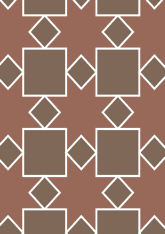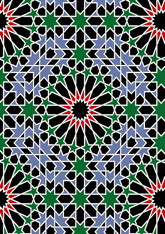The number in this group is 75, which is 13% of the total. Another question is needed - for this see page.
The second group are examples of patterns which have the 90 degree star, but not the 45 degree one. An example of two of these are: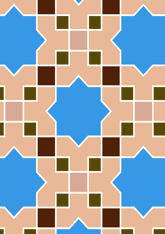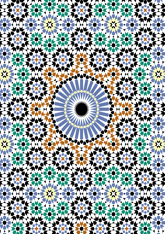The number in this group is 414, which is 73% of the total. Another question is needed - for this see page.
The last group are examples of patterns which have both of the two types of stars. An example of two of these are: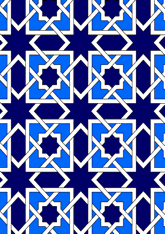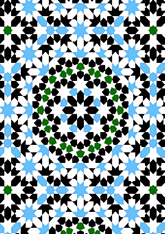The number in this group is 76, which is 13% of the total. Another question is needed - for this see page.

v53

Tiling Search was created by Brian Wichmann, and is maintained by MIT Libraries, through support from the Aga Khan Documentation Center.

Also supported by Performant Software Solutions LLC.